Courses

# P. Bahadur Test: Redox Reactions & Electro Chemistry

## 25 Questions MCQ Test Chemistry for JEE | P. Bahadur Test: Redox Reactions & Electro Chemistry

Description
This mock test of P. Bahadur Test: Redox Reactions & Electro Chemistry for JEE helps you for every JEE entrance exam. This contains 25 Multiple Choice Questions for JEE P. Bahadur Test: Redox Reactions & Electro Chemistry (mcq) to study with solutions a complete question bank. The solved questions answers in this P. Bahadur Test: Redox Reactions & Electro Chemistry quiz give you a good mix of easy questions and tough questions. JEE students definitely take this P. Bahadur Test: Redox Reactions & Electro Chemistry exercise for a better result in the exam. You can find other P. Bahadur Test: Redox Reactions & Electro Chemistry extra questions, long questions & short questions for JEE on EduRev as well by searching above.
QUESTION: 1

### According to Faraday’s Second Law of Electrolysis the amounts of different substances liberated by the same quantity of electricity passing through the electrolytic solution are proportional:

Solution:

The amounts of different substances liberated by the same quantity of electricity passing through the electrolytic solution are proportional to their chemical equivalent weights.

QUESTION: 2

### Charge on one mole of electrons i.e. one Faraday is approximately:

Solution:

Charge on one electron is 1.6 x 10-19 C.
1 mole of electron 6.023 x 1023 electrons.
So charge on one mole of electron = 1.6 x 10-19 C x 6.023 x 1023 = 96500 C mol-1

QUESTION: 3

### In the primary batteries,

Solution:

Primary batteries cannot be recharged and reused.

QUESTION: 4

A secondary cell after use ________ recharged by passing current through it in the ________ so that it can/can’t be used again.

Solution:

Secondary cell can be recharged and reused.

QUESTION: 5

Fuel cells are _________ that are designed to convert the energy of combustion of fuels like hydrogen, methane, methanol, etc. directly into _________.

Solution:
• fuel cell is an electrochemical cell that converts the chemical energy of a fuel (often hydrogen) and an oxidizing agent (often oxygen) directly into electrical energy through a pair of redox reactions.
• Fuel cells are different from most batteries in requiring a continuous source of fuel and oxygen (usually from air) to sustain the chemical reaction, whereas in a battery the chemical energy usually comes from metals and their ions or oxides that are commonly already present in the battery, except in flow batteries.
• Fuel cells can produce electricity continuously for as long as fuel and oxygen are supplied.

Setup of a Fuel cell: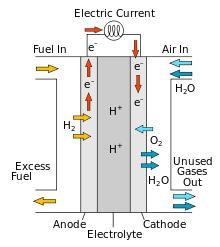QUESTION: 6

In corrosion, a metal is _________ of electrons to oxygen and results in the formation of oxides.

Solution:

In Corrosion, a metal is oxidized by loss of electrons and results in the formation of oxides.

• Corrosion is a natural process that converts a refined metal into a more chemically stable form such as oxide, hydroxide, or sulfide.
• It is the gradual destruction of materials (usually a metal) by chemical and/or electrochemical reaction with their environment.
• Corrosion engineering is the field dedicated to controlling and preventing corrosion.
QUESTION: 7

One of the simplest methods of preventing corrosion is to prevent the surface of the metallic object to come in contact with atmosphere. This can be done:

Solution:

The best way to prevent corrosion is to prevent the surface of the metallic objects to come in contact with the atmosphere.

• The rusting of iron can be prevented by painting, oiling, greasing or varnishing its surface.
• Galvanization is another method of protecting iron from rusting by coating iron with a thin layer of zinc.
• Corrosion of iron is prevented by coating iron with non-xcorrosive substance like carbon. This process is termed as alloying .
QUESTION: 8

An electrochemical method is to provide a sacrificial electrode of another metal (like Mg, Zn, etc.) which corrodes itself but saves the object. A typical example is

Solution:

Galvanization is a process in which iron is coated with reactive metal like Zn which get corroded hence preventing iron from corrosion.

Electro-Galvanization: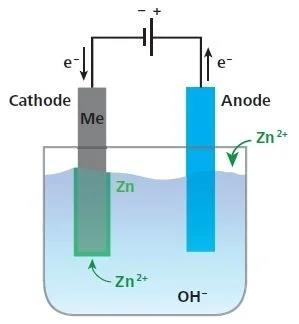QUESTION: 9

Correct arrangement of Al, Cu, Fe, Mg and Zn in the order in which they displace each other from the solution of their salts is

Solution:

A more reactive metal displaces a less reactive metal from the salt solution (refer to reactivity series).

The order in which metals displace each other from the solution of their salts can be given with the help of their standard electrode potential. Since magnesium has the least standard electrode potential so it is the most strong reducing agent.

So the required order we get is: Mg > Al > Zn > Fe > Cu

Reactivity Series:QUESTION: 10

The standard emf of galvanic cell involving 3 moles of electrons in its redox reaction is 0.59 V. The equilibrium constant for the reaction of the cell is

Solution:

The standard emf of a galvanic cell involving 3 moles of electrons in its redox reaction is 0.59V.

cell​ = 0.59V
n=3
The equilibrium constant for the reaction of the cell is given by the expression: ln K = RT nFEºcell
ln K = 8.314 × 298 × 3 × 96500 × 0.59 = 68.9
K≈1030

The equilibrium constant for the reaction of the cell is 1030.

QUESTION: 11

Emf of the cell

Mg (s) | Mg2+ (0.001M) || Cu2+ (0.0001M) | Cu(s)   at 298 K is

Solution: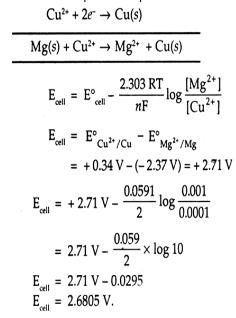QUESTION: 12

Emf of the cell

Fe (s) | Fe2+ (0.001 M)|| H+ (1M)|H2 (g) (1 bar) |Pt(s)   at 298 K is

(Given: Eocell ​= +0.44 V)

Solution: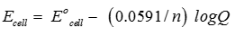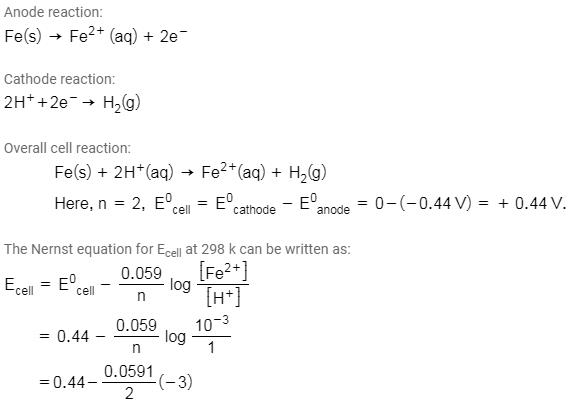⇒Ecell ​= 0.44 + 0.089V

⇒Ecell​ = 0.53V

QUESTION: 13

The conductivity of 0.20 M solution of KCl at 298 K is 0.0248 S  cm-1. Calculate its molar conductivity.

Solution:

k (Conductivity) =0.0248 Scm−1, C (molarity) = 0.20 M, T (temperature) = 298 K
Λm​ = ? (molar conductivity)

Λm​ = (1000×k​) / C = (1000×0.0248) / 0.20 ​= 124 S cm2 mol−1

The molar conductivity is 124 S cm2 mol−1

QUESTION: 14

The resistance of a conductivity cell containing 0.001M KCl solution at 298 K is 1500 Ω. What is the cell constant if conductivity of 0.001M KCl solution at 298 K is 0.146 × 10-3 S cm-1.

Solution:

Κ = G x cell constant and G = 1/R.

QUESTION: 15

How much charge is required for the reduction of 1 mol of Al3+ to Al?

Solution:

For reduction of 1 mol of Al3+ to Al, 3 mol of electrons are required so total charge will be 3F.

QUESTION: 16

How much electricity in terms of Faraday is required to produce 40.0 g of Al from molten  Al2O3?

Solution:

Moles of Al = 40/27. For 1 mol of Al deposition 3F charge is required.

QUESTION: 17

Three electrolytic cells A, B, C containing solutions of ZnSO4, AgNO3 and CuSO4, respectively are connected in series. A steady current of 1.5 amperes was passed through them until 1.45 g of silver deposited at the cathode of cell. How long did the current flow? What mass of copper and zinc were deposited?

Solution: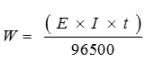And mass of Zn and Cu deposited will be in ratio of their equivalent mass.

QUESTION: 18

The standard emf of galvanic cell involving 3 moles of electrons in its redox reaction is 0.59 V. The equilibrium constant for the reaction of the cell is

Solution: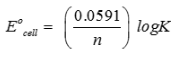QUESTION: 19

An increase in equivalent conductance of a strong electrolyte with dilution is mainly due to

Solution:

Equivalent conductance increases on dilution for a strong electrolyte because of increase in mobility of ions.

QUESTION: 20

For the reduction of silver ions with copper metal the standard cell potential was found to be +0.46V at 25°C. The value of standard Gibbs energy, ΔG° wll be (F = 96500 C mol-1)

Solution: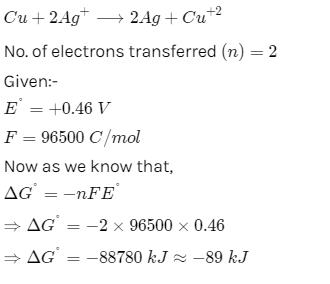QUESTION: 21

Which of the following electrolytic solutions has the least specific conductance?

Solution:

Specific conductance decreases with dilution because of decrease in the number of ions per unit volume.

QUESTION: 22

The highest electrical conductivity of the following aqueous solutions is of

Solution:

Acidity increases on attaching electron withdrawing group because of stability of conjugate base.

QUESTION: 23

The standard electrode potential is measured by

Solution:

Voltmeter measures the potential.

QUESTION: 24

When KMnO4 acts as an oxidizing agent and ultimately forms MnO42-, MnO2, Mn2O3, and Mn2+ then the number of electrons transferred in each case

Solution:

No. of electrons = change in oxidation number of active elemnt.

QUESTION: 25

On the basis of the following E° values, the strongest oxidizing agent is: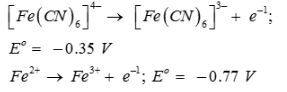Solution:
• The strongest oxidizing agent is one having more positive or less negative reduction potential.
• For the strongest oxidizing agent, the oxidizing potential should be the least.

Here, the oxidizing potential of Fe+2 is less than that of [Fe(CN)6​]4−. Therefore, Fe+2 is a stronger oxidizing agent than [Fe(CN)6​]4−.

• Also, the stronger oxidizing agent should easily reduce itself.

Here, Fe+3 is easily reduced than Fe+2. Therefore, among all the four, Fe+3 is the stronger oxidizing agent.